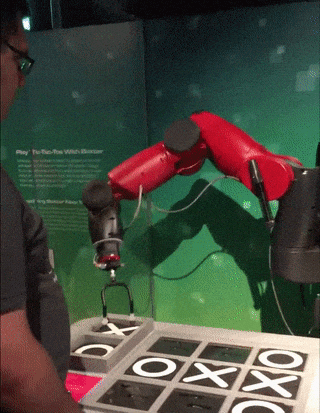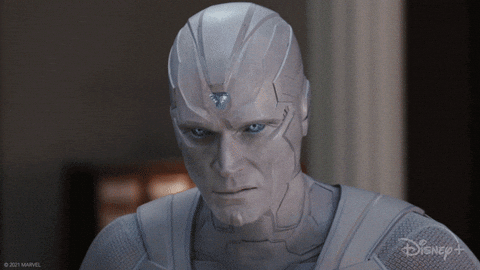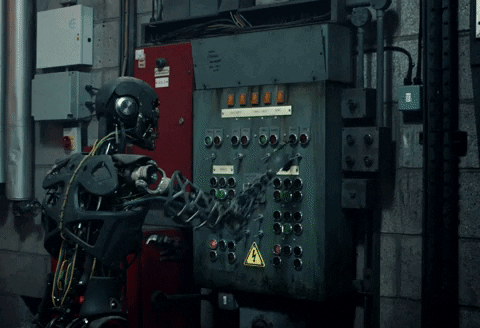﻿ Let's fight against the machines! | InterSystems Developer Community | AI
Article
Henry Pereira · Aug 2, 2021 8m read

# Let's fight against the machines!Easy, easy, I'm not promoting a war against the machines in the best sci-fi way to avoid world domination of Ultron or Skynet.
Not yet, not yet 🤔

I invite you to challenge the machines through the creation of a very simple game using ObjectScript with embedded Python.

I have to say that I got super excited with the feature of Embedded Python on InterSystems IRIS, it's incredible the bunch of possibilities that opens to create fantastic apps.

Let's build a tic tac toe, the rules are quite simple and I believe that everyone knows how to play.

That's what saved me of the tedium in my childhood during long car trips with family before chidren have cellphones or tablets, nothing like challenge my siblings to play some matches on the blurry glass.

So buckle up and let's go!

## Rules

As said, the rules are quite simple:

• only 2 players for set
• it's played in turns in a grid of 3x3
• the human player will always be the letter X and the computer the letter O
• the players will only be able to put the letters in the empty spaces
• the first to complete a sequence of 3 equal letters on the horizontal, or on vertical or on diagonal, is the winner
• when the 9 spaces are occupied that will be draw and the end of the matchAll the mechanism and the rules we will write on ObjectScript, the mechanism of the computer player will be written in Python.

## Let's get the hands dirty

We will control the board in a global, in wich each row will be in a node and each column in a piece.

Our first method is to initiate the board, to make it easy I will initiate the global already with the nodes(rows A, B and C) and with the 3 pieces:

``````/// Iniciate a New Game
ClassMethod NewGame() As %Status
{
Set sc = \$\$\$OK
Kill ^TicTacToe
Set ^TicTacToe("A") = "^^"
Set ^TicTacToe("B") = "^^"
Set ^TicTacToe("C") = "^^"
Return sc
}
``````

at this moment we will create a method to add the letters in the empty spaces, for this each player will give the location of the space on the board.

Each row a letter and each column a number, to put the X in the middle, for example, we pass B2 and the letter X to the method.

``````ClassMethod MakeMove(move As %String, player As %String) As %Boolean
{
Set \$Piece(^TicTacToe(\$Extract(move,1,1)),"^",\$Extract(move,2,2)) = player
}
``````

Let's validate if the coordination is valid, the most simple way I see is using a regular expression:

``````ClassMethod CheckMoveIsValid(move As %String) As %Boolean
{
Set regex = ##class(%Regex.Matcher).%New("(A|B|C){1}[0-9]{1}")
Set regex.Text = \$ZCONVERT(move,"U")
Return regex.Locate()
}
``````

we need to garantee that the selected space is empty

``````ClassMethod IsSpaceFree(move As %String) As %Boolean
{
Quit (\$Piece(^TicTacToe(\$Extract(move,1,1)),"^",\$Extract(move,2,2)) = "")
}
``````

Nooice!

Now let's check if any player won the set or if the game is already finished, for this let's create the method CheckGameResult.

First we check if there was any winner completing by the horizontal, we will use a list with the rows and a simple \$Find solves

``````    Set lines = \$ListBuild("A","B","C")
// Check Horizontal
For i = 1:1:3 {
Set line = ^TicTacToe(\$List(lines, i))
If ((\$Find(line,"X^X^X")>0)||(\$Find(line,"O^O^O")>0)) {
Return \$Piece(^TicTacToe(\$List(lines, i)),"^", 1)_" won"
}
}
``````

With another For we check the vertical

``````For j = 1:1:3 {
If ((\$Piece(^TicTacToe(\$List(lines, 1)),"^",j)'="") &&
(\$Piece(^TicTacToe(\$List(lines, 1)),"^",j)=\$Piece(^TicTacToe(\$List(lines, 2)),"^",j)) &&
(\$Piece(^TicTacToe(\$List(lines, 2)),"^",j)=\$Piece(^TicTacToe(\$List(lines, 3)),"^",j))) {
Return \$Piece(^TicTacToe(\$List(lines, 1)),"^",j)_" won"
}
}
``````

to check the diagonal:

``````    If ((\$Piece(^TicTacToe(\$List(lines, 2)),"^",2)'="") &&
(
((\$Piece(^TicTacToe(\$List(lines, 1)),"^",1)=\$Piece(^TicTacToe(\$List(lines, 2)),"^",2)) &&
(\$Piece(^TicTacToe(\$List(lines, 2)),"^",2)=\$Piece(^TicTacToe(\$List(lines, 3)),"^",3)))||
((\$Piece(^TicTacToe(\$List(lines, 1)),"^",3)=\$Piece(^TicTacToe(\$List(lines, 2)),"^",2)) &&
(\$Piece(^TicTacToe(\$List(lines, 2)),"^",2)=\$Piece(^TicTacToe(\$List(lines, 3)),"^",1)))
)) {
Return ..WhoWon(\$Piece(^TicTacToe(\$List(lines, 2)),"^",2))
}
``````

at last, we check if there was a draw

``````    Set gameStatus = ""
For i = 1:1:3 {
For j = 1:1:3 {
Set:(\$Piece(^TicTacToe(\$List(lines, i)),"^",j)="") gameStatus = "Not Done"
}
}
Set:(gameStatus = "") gameStatus = "Draw"
``````

Great!

## It's time to build the machine

Let's create our opponent, we need to create an algorithm able to calculate all the available movements and use a metric to know wich is the best movement.

The ideal is to use an algorithm of decision called MiniMax (Wikipedia: MiniMax)The MiniMax algorithm is a decision rule used in games theory, decision theory and artificial intelligence.

Basicaly, we need to know how to play assuming wich will be the possible movements of the opponent and catch the best scene possible.

In details, we take the actual scene and recursively check the result of the movement of each player, in case the computer wins the match we score with +1, in case it looses we then score with -1 and 0 if draw.

If it is not the end of the game, we open another tree with the current game state. After that, we find the move with the maximum value to the computer and the minimum to the opponent.

See the diagram below, there are 3 available movements: B2, C1 and C3.

Choosing C1 or C3, the opponent has a chance to win in the next turn, but if choosing B2 dosen't matter the movement the opponent chooses, the machine wins the match.It's like have the time stone in our hands and try to find the best timeline.Converting to python

``````ClassMethod ComputerMove() As %String [ Language = python ]
{
import iris
from math import inf as infinity
computerLetter = "O"
playerLetter = "X"

def isBoardFull(board):
for i in range(0, 8):
if isSpaceFree(board, i):
return False
return True

def makeMove(board, letter, move):
board[move] = letter

def isWinner(brd, let):
# check horizontals
if ((brd == brd == brd == let) or \
(brd == brd == brd == let) or \
(brd == brd == brd == let)):
return True
# check verticals
if ((brd == brd == brd == let) or \
(brd == brd == brd == let) or \
(brd == brd == brd == let)):
return True
# check diagonals
if ((brd == brd == brd == let) or \
(brd == brd == brd == let)):
return True
return False

def isSpaceFree(board, move):
if(board[move] == ''):
return True
else:
return False

def copyGameState(board):
dupeBoard = []
for i in board:
dupeBoard.append(i)
return dupeBoard

def getBestMove(state, player):
done = "Done" if isBoardFull(state) else ""
if done == "Done" and isWinner(state, computerLetter): # If Computer won
return 1
elif done == "Done" and isWinner(state, playerLetter): # If Human won
return -1
elif done == "Done":    # Draw condition
return 0

# Minimax Algorithm
moves = []
empty_cells = []
for i in range(0,9):
if state[i] == '':
empty_cells.append(i)

for empty_cell in empty_cells:
move = {}
move['index'] = empty_cell
new_state = copyGameState(state)
makeMove(new_state, player, empty_cell)

if player == computerLetter:
result = getBestMove(new_state, playerLetter)
move['score'] = result
else:
result = getBestMove(new_state, computerLetter)
move['score'] = result

moves.append(move)

# Find best move
best_move = None
if player == computerLetter:
best = -infinity
for move in moves:
if move['score'] > best:
best = move['score']
best_move = move['index']
else:
best = infinity
for move in moves:
if move['score'] < best:
best = move['score']
best_move = move['index']

return best_move

lines = ['A', 'B', 'C']
game = []
current_game_state = iris.gref("^TicTacToe")

for line in lines:
for cell in current_game_state[line].split("^"):
game.append(cell)

cellNumber = getBestMove(game, computerLetter)
next_move = lines[int(cellNumber/3)]+ str(int(cellNumber%3)+1)
return next_move
}
``````

First I convert the global in a simple array, ignoring columns and rows, leting flat to facilitate.

At each analised move we call the method copyGameState that, as the name says, copys the state of the game in that moment, where we apply the MiniMax.

The method getBestMove that will be called recursevely until ends the game finding a winner or draw.

First the empty spaces are mapped and we verify the result of each move, changing between the players.

The results are stored in move['score'] to, after we check all the possibilities, find the best move.

I hope you have had fun, it is possible to improve the intelligence using algorithms like Alpha-Beta Pruning(Wikipedia: AlphaBeta Pruning) or neural network, just take care not to give life to Skynet.Feel free to leave any comments or questions.

That's all folks

Complete source code:
InterSystems Iris version 2021.1.0PYTHON

41
5 768
Discussion (8)3
PS: Would the best timeline, the sacred timeline?!Very nice, @Henry Pereira !